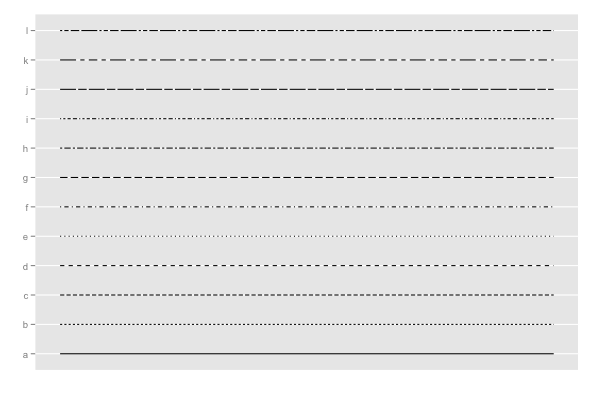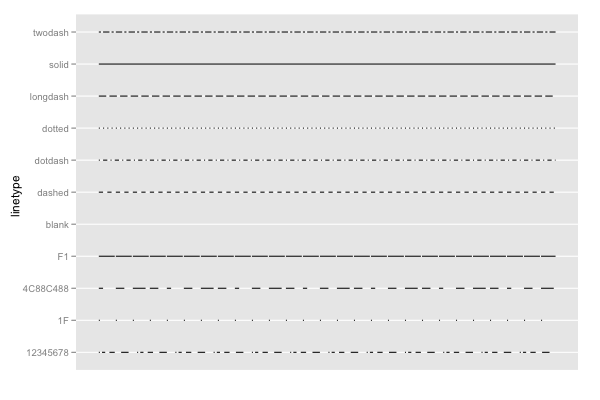## ggplot2 Quick Reference: linetype

Geoms that draw lines have a "linetype" parameter.

Legal values are the strings "blank", "solid", "dashed", "dotted", "dotdash", "longdash", and "twodash". Alternatively, the numbers 0 to 6 can be used (0 for "blank", 1 for "solid", ...). Moreover, one can also use strings that define the linetype with up to 8 hexadecimal digits (each digit specifying the length of interleaved lines and gaps).

For most geoms, the default linetype is 1 (a solid line).

The linetype can be set to a constant value or it can be mapped via a scale.

### Setting to constant value

To set the linetype to a constant value, use the linetype geom parameter (e.g., `geom_line(data=d, mapping=aes(x=x, y=y), linetype=3)` sets the linetype of all lines in the layer to 3, which corresponds to a dotted line).

### Mapping via scale_linetype_discrete

The `scale_linetype_discrete` scale maps up to 12 distinct values to 12 pre-defined linetypes.```d=data.frame(a=c("a","b","c","d","e","f","g","h", "i", "j", "k", "l"))
ggplot() +
scale_x_continuous(limits=c(0,1), breaks=NA, name="") +
scale_y_discrete(name="") +
scale_linetype_discrete(legend=F) +
geom_segment(data=d, mapping=aes(x=0, xend=1, y=a, yend=a, linetype=a))```

### Mapping via scale_linetype_identity

The `scale_linetype_identity` scale can be used to pass through any legal linetype value (its mapping is the identity function, and thus it does not change anything).```d=data.frame(lt=c("blank", "solid", "dashed", "dotted", "dotdash", "longdash", "twodash", "1F", "F1", "4C88C488", "12345678"))
ggplot() +
scale_x_continuous(name="", limits=c(0,1), breaks=NA) +
scale_y_discrete(name="linetype") +
scale_linetype_identity() +
geom_segment(data=d, mapping=aes(x=0, xend=1, y=lt, yend=lt, linetype=lt))```

### Note

The ggplot2 `linetype` parameter corresponds to the `lty` parameter of the R base graphics package (see the "lty" description on the help page of the par() function).